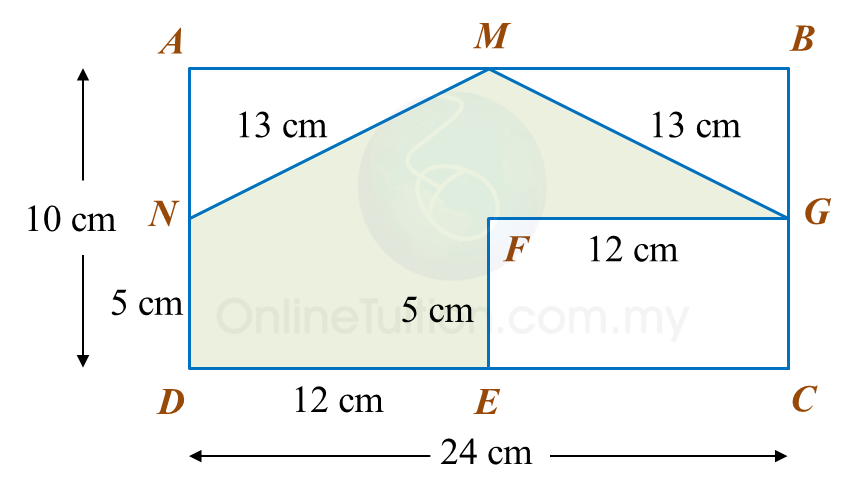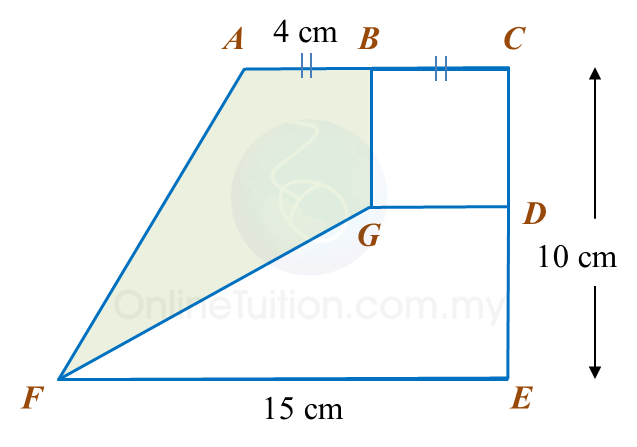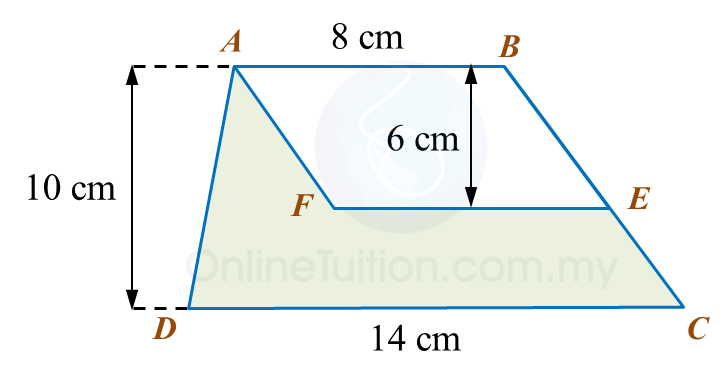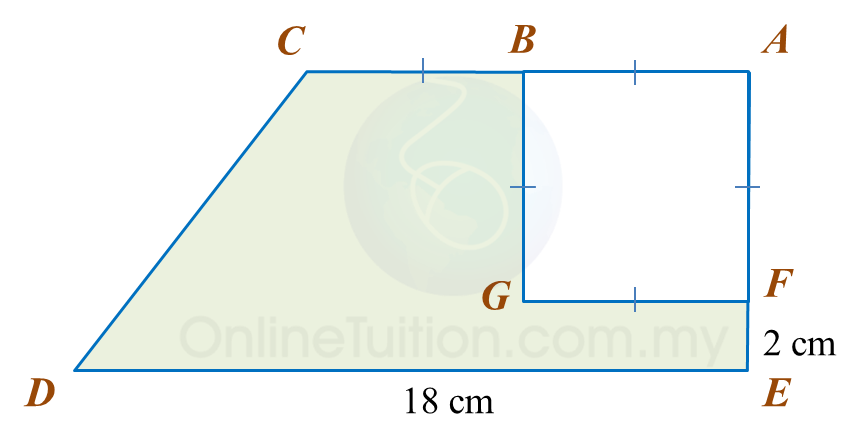# 11.2.3 Perimeter and Area, PT3 Practice

     
Question 9:
 
In diagram below, ADB is a right-angled triangle and DBFE is a square. C is the midpoint of DB and CH = CD.Calculate the area, in cm2, of the coloured region.
 

 Solution:
     
 
 
 
      
Question 10:
 
Diagram below shows a rectangle ACDE.Calculate the area, in cm2, of the coloured region.
 

 Solution:
     
 
 
 
Question 11:
Diagram below shows a sketch of parallelogram shaped garden, PQRS that consists of flower beds and a playground.Calculate the area, in m2, of the flower beds.

Solution:

# 11.2.2 Perimeter and Area, PT3 Practice

                    
Question 5:
 
In diagram below, PQUV is a square, QRTU is a rectangle and RST is an equilateral triangle.The perimeter of the whole diagram is 310 cm.
 Calculate the length, in cm, of PV.
 
 Solution:
                               
 
 
 
                    
Question 6:
 
In diagram below, ABCD and CGFE are rectangles. M, G, E and N are midpoints of AB, BC, CD and DA respectively.Calculate the perimeter, in cm, of the coloured region.
 
 Solution:Question 7:
 Diagram below shows a trapezium BCDE and a parallelogram ABEF. ABC and FED are straight lines.The area of ABEF is 72 cm2.
 Calculate the area, in cm2, of trapezium BCDE.
 
 Solution:
        

 
 
      
Question 8:
 In diagram below, ACEF is a trapezium and BCDG is a square.Calculate the area, in cm2, of the coloured region.
 
 Solution:
     

 
 

# 11.2.1 Perimeter and Area, PT3 Practice

Question 1:
In the diagram, ABCD is a trapezium and ABEF is a parallelogram.Calculate the area, in cm2, of the coloured region.

Solution
:

Question 2:
Diagram below shows a rectangle ABCD.Calculate the area, in cm2, of the coloured region.

Solution:

Question 3:
In diagram below, AEC is a right-angled triangle with an area of 54 cm2 and BCDF is a rectangle.Calculate
(a) the perimeter, in cm, of the coloured region.
(b) the area, in cm2, of the coloured region.

Solution:

Question 4:
Diagram below shows a trapezium ABCDE. ABGF is a square with an area of 36 cm2.Calculate
(a) the perimeter, in cm, of the coloured region.
(b) the area, in cm2, of the coloured region.

Solution: